Company Analysis

FBN Holdings 2019Q1: Strong Deposits, Huge Assets, Fettered By High NPL Ratio

Company: FBN Holdings Plc
Rating: Hold
Current Market Price: N7.40
NPL RATIO (Q1-2019): 25.3%
Year High: N9.00
Year Low: N7.00
Fair Value: N10.11
Equity Analyst: Jeariogbe Tunde Segun

• This report observes the financial performance indices of FBN Holdings for the period ended March 31, 2018; compares same with released statistics for the similar period in 2018 to establish growth. Or otherwise.
• Non-Performing Loan (NPL) ratio is currently 25.30% of gross loans as at the end of March 2019, slightly down from 25.90% at year-end 2018.
• The Loan growth status at the end of the period under review is quite worrisome, as it will require higher loan-loss provisions to achieve reasonable adjustment, a situation that will negatively impact the group’s profitability/ratios.
• Nonetheless, in our opinion, FBNH Holdings looks very strong on key parameters such as deposits, and asset base, amongst others. Should the management achieve improvement via a healthy/significant lowering in its high NPL ratio, we foresee an equally robust recovery of its financial performance.
• We have, therefore, fairly valued its shares at N10.11 and rated it a Hold in the expectation that the management of FBNH will wake up an strengthen the risk management framework of its operating subsidiary- First Bank of Nigeria Limited while embarking of loan recovery. These would be for the ultimate good of investors and shareholders who will increase their appetite for units of the stock, leading to capital appreciations and enhanced returns for all stakeholders.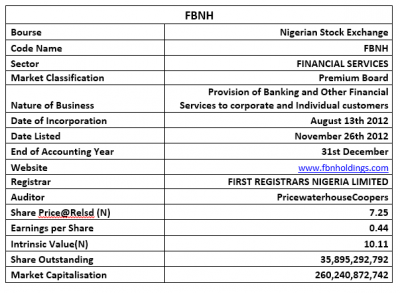Company figures
• In the period under review, gross earnings improved over the comparable period by 6.61%, rising from N130.63 billion to N139.27 billion.
• Of this amount, interest income stood at N112.04 billion, as against the N110.89 billion reported in the similar period of 2018.
• Interest Expenses inched 7.73% up, to N37.86 billion as against the N35.15 billion reported in the corresponding quarter of 2018.
• Net Interest Income, therefore, stood at N1.95 billion, 41.67% lower than the N3.35 billion achieved in the corresponding period of 2018.
• Operating Expenses appreciated by 27.82% over the reported figure in 2018Q1 financial performance. Please understand that this index is currently estimated at N65.51 billion, as against N51.25 billion
• Profit before Tax for the period is estimated at N19.30 billion, just 2.59% above the N18.81 billion achieved in the similar period of 2018.
• Having considered Tax Expense, Net Profit reported for the period stood at N15.79 billion, just 6.93% better than the N14.76 billion earned through the first three months of 2018.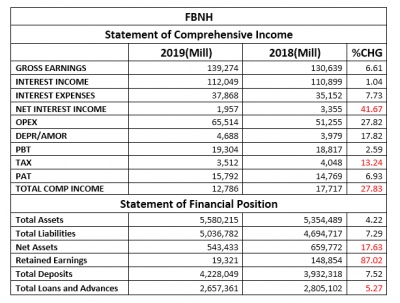• Total Assets valuation improved by a marginal 4.22% to N5.58 trillion, compared to the N5.35 trillion assets valuation at the end of 2018 first quarter business.
• Similarly, Total Liabilities grew by 7.29% to stand at N5.03 trillion against the N4.69 trillion reported in 2018
• Net Assets dipped to N543.43 billion, as against N659.77 billion in 2018. The difference between both figures is the same as a drop of 17.63%.
• Similarly, retained earnings slipped to N19.32 billion, standing 87.02% below the N148.85 trillion reported in the similar period of 2018.
• Total Deposits achieved through the first three months of 2019 grew marginally by 7.52% to N4.22 trillion, compared to N3.93 trillion in 2018 first quarter.
• Total Loans and Advances adjusted down by 5.27% to stand at N2.65 trillion as against the N2.80 trillion recorded in the corresponding period of 2018.

Financial Strength/Solvency Ratios
• Debt Ratio, which compares Total Assets to Total Liabilities, stood at 0.9x or 90%. This implies that Total Liabilities is 90% of Total Assets. (Please understand that Total Liabilities is inclusive of Total Deposits for the period.)
• Total Debt to Equity for the quarter stood at 9.27x, same as 30.25% above the 7.12x estimated in 2018. This implies that the company used 9.27x of its equity through the period (note that debt, in this case, is total liabilities, and includes total deposits for the period).
• Equity Ratio which compares Equity to Total Assets, stood at 0.10x as against 0.12x, implying that the reported Equity value at the end of the period is 10% of FBNH’s Total Assets, which signifies a reduction from the previously estimated 12%.
• Estimated Beta value for FBNH as at the time this report was compiled stood at 1.04, which is above market beta but below the industry average for the review period.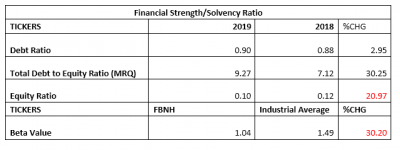Profitability Ratios
• EBITDA margin estimated for the period stood at 7.29%, which was 16.30% below the 8.71% estimated from the corresponding quarters’ numbers.
• Pre-Tax Margin dipped by slight 3.77% to 13.86%, as against the 14.40% estimated at the end of 2018 first quarter.
• Interest Expenses to Gross Earnings inched up by 1.05% to 27.19% against the previously estimated 26.91%.
• Return on Average Equity through the first three months of 2019 was 2.91%, compared to the previous 2.24%
• See table below for detailed profitability ratios and comparisons.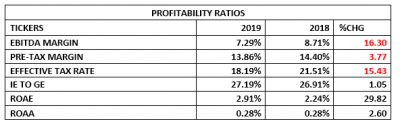Efficiency Ratios
• Operating Expenses at the end of the period is estimated at 47.04% of the estimated Gross Earnings, this is higher than the 39.23% seen in 2018 first quarter financials. Please note that this implies an increased rate of expenses.
• Gross Earnings estimated from the bank financials is same as 2.50% of the Total Assets value posted for the period under analysis.
• Total Loan and Advances for the quarter is 62.85% of Total Debt, which is lower when compared to 71.33% estimate of 2018 first quarter.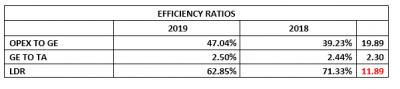Investment/Valuation Ratios
• The profit earned per unit of FBNH is estimated at N0.44, a slight increase over the N0.41 earned in 2018.
• Total Comprehensive Income/share is estimated at N0.36, this is 27.83% below the N0.49 TCI/share achieved in 2018. Two items responsible for the difference are the unrealized net gains/(losses) arising during the period and Exchange rate difference on the translation of foreign operations.
• P/E-Ratio reduced to 4.12x from 6.96x, which implies reduced investor sentiments for the shares of FBNH, while also confirming a faster investment recovery period.
• The earnings per share represented a Yield of 6.07% of the price of each FBNH share on the floor of the exchange on the day the result was unveiled to the investing public.
• The estimated value of each unit in its book is N15.14, which dropped by 17.63% from the previous estimate of N18.38.
• Confirming the academic under-priced stand of FBNH shares on the floor of the exchange is the Price to Book Value estimate at below unity.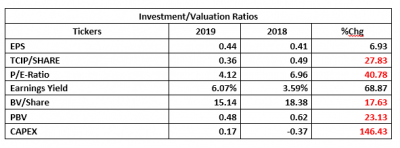Valuation
Assuming a constant growth rate of the current dividend of FBNH, the constant perpetual growth rate of the Gordon Growth Model was put to use. Also, assuming our discount rate at 11% and making use of the estimated sustainable growth rate; we, therefore, arrived at N10.11 valuation for each unit of FBNH. Thus, we have rated FBNH a Hold.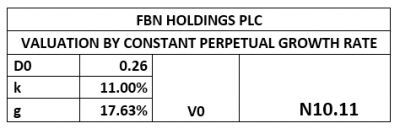Check Also
Close